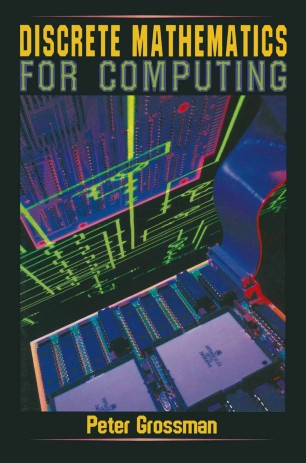# Discrete Mathematics for Computing

• Peter GrossmanTextbook

1. Front Matter
Pages i-xiii
2. Peter Grossman
Pages 1-13
3. Peter Grossman
Pages 14-27
4. Peter Grossman
Pages 28-43
5. Peter Grossman
Pages 44-68
6. Peter Grossman
Pages 69-91
7. Peter Grossman
Pages 92-108
8. Peter Grossman
Pages 109-132
9. Peter Grossman
Pages 133-157
10. Peter Grossman
Pages 158-169
11. Peter Grossman
Pages 170-196
12. Peter Grossman
Pages 197-214
13. Peter Grossman
Pages 215-236
14. Peter Grossman
Pages 237-259
15. Back Matter
Pages 260-289

### Introduction

Written in a clear and informal style aimed especially at students with a limited background in mathematics, this introduction to discrete mathematics presents the material that forms the essential mathematical background for studies in computing and information systems. The topics covered include number systems, logic, relations, functions, induction, recursion, Boolean algebra, combinatorics, graph theory and number theory. The text contains many examples of applications of the theory to problems in computing, including a chapter on the representation and manipulation of numbers in a computer, examples of the use of propositional logic in program design, a section discussing the relationship between the mathematical and programming concepts of a function, and a chapter on the estimation of the time complexity of algorithms. A feature of the book is the use of algorithms written in pseudo code in many examples throughout the text. The exercises at the end of each chapter provide both routine drill of basic techniques and more challenging problems designed to enhance and extend the students' understanding. Answers to most of the exercises are provided at the back of the book. With its emphasis on presenting mathematical concepts clearly and simply, without compromising on mathematical correctness, and with its frequent use of examples drawn from computing, Discrete Mathematics for Computing is suitable for use as a text for a one-semester introductory course in discrete mathematics, particularly for students who are undertaking studies in computing and information systems.

### Keywords

algebra algorithms Boolean algebra code combinatorics complexity computational complexity discrete mathematics graph theory information logic Mathematica mathematics number theory programming

#### Authors and affiliations

• Peter Grossman
• 1
1. 1.Department of MathematicsMonash UniversityAustralia